Test: Basic Nodal & Mesh Analysis - 1

# Test: Basic Nodal & Mesh Analysis - 1

Test Description

## 10 Questions MCQ Test GATE Electrical Engineering (EE) 2023 Mock Test Series | Test: Basic Nodal & Mesh Analysis - 1

Test: Basic Nodal & Mesh Analysis - 1 for Electrical Engineering (EE) 2023 is part of GATE Electrical Engineering (EE) 2023 Mock Test Series preparation. The Test: Basic Nodal & Mesh Analysis - 1 questions and answers have been prepared according to the Electrical Engineering (EE) exam syllabus.The Test: Basic Nodal & Mesh Analysis - 1 MCQs are made for Electrical Engineering (EE) 2023 Exam. Find important definitions, questions, notes, meanings, examples, exercises, MCQs and online tests for Test: Basic Nodal & Mesh Analysis - 1 below.
Solutions of Test: Basic Nodal & Mesh Analysis - 1 questions in English are available as part of our GATE Electrical Engineering (EE) 2023 Mock Test Series for Electrical Engineering (EE) & Test: Basic Nodal & Mesh Analysis - 1 solutions in Hindi for GATE Electrical Engineering (EE) 2023 Mock Test Series course. Download more important topics, notes, lectures and mock test series for Electrical Engineering (EE) Exam by signing up for free. Attempt Test: Basic Nodal & Mesh Analysis - 1 | 10 questions in 30 minutes | Mock test for Electrical Engineering (EE) preparation | Free important questions MCQ to study GATE Electrical Engineering (EE) 2023 Mock Test Series for Electrical Engineering (EE) Exam | Download free PDF with solutions
 1 Crore+ students have signed up on EduRev. Have you?
Test: Basic Nodal & Mesh Analysis - 1 - Question 1

### Of the two methods of loop and node variable analysis

Test: Basic Nodal & Mesh Analysis - 1 - Question 2

### Which of the following is ​​​​true about the circuit shown below?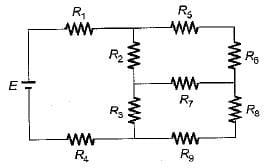Detailed Solution for Test: Basic Nodal & Mesh Analysis - 1 - Question 2

Number of junction point for the given circuit is 6.

Test: Basic Nodal & Mesh Analysis - 1 - Question 3

### For the circuit shown below, the current through the 1 Ω resistor is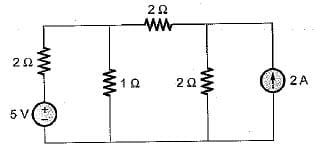Detailed Solution for Test: Basic Nodal & Mesh Analysis - 1 - Question 3

Using source transformation, the current source is converted to a voltage source as shown below.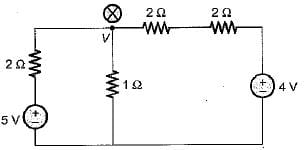Applying nodal analysis at node (x), we get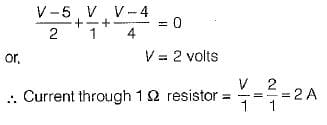Test: Basic Nodal & Mesh Analysis - 1 - Question 4

An electric circuit with 8 branches and 4 nodes will have

Detailed Solution for Test: Basic Nodal & Mesh Analysis - 1 - Question 4

Number of loop equation
= b - ( n - 1)
= 8 - ( 4 - 1 )
= 8 - 3 = 5

Test: Basic Nodal & Mesh Analysis - 1 - Question 5

For the circuit shown below, the current through the 10 V battery is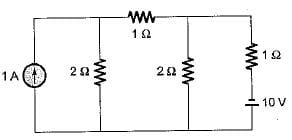Detailed Solution for Test: Basic Nodal & Mesh Analysis - 1 - Question 5

Using source transformation, first we convert the current source into an equivalent voltage source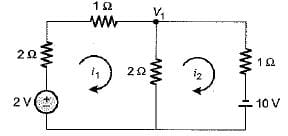Applying KVL in loop-1, we get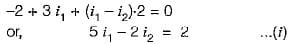Applying KVL in loop-2, we get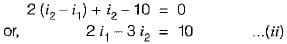On solving equations (i) and (//). we get

i1 = 2.36 A and i2 = 4.91 A

Hence, the current through the battery of 10 V is

i2 = 4.91 A

Test: Basic Nodal & Mesh Analysis - 1 - Question 6

Find the value of the currents I1 and I2 flowing clockwise in the first and second mesh respectively.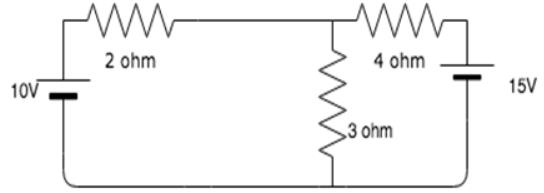Detailed Solution for Test: Basic Nodal & Mesh Analysis - 1 - Question 6

The two mesh equations are:
5I1 - 3I2 = 10
-3I1 + 7I2 = -15
Solving the equations simultaneously, we get I1 = 0.96A and I2 = -1.73A.

Test: Basic Nodal & Mesh Analysis - 1 - Question 7

The value of V in volts for the circuit shown below is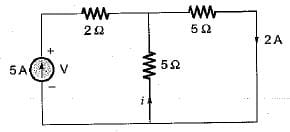Detailed Solution for Test: Basic Nodal & Mesh Analysis - 1 - Question 7

The equivalent resistance across the 5 A current source is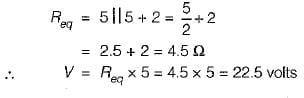Test: Basic Nodal & Mesh Analysis - 1 - Question 8

The number of KVL and KCL equations for the circuit shown below are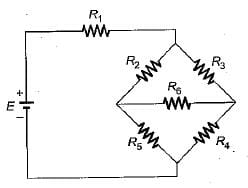Detailed Solution for Test: Basic Nodal & Mesh Analysis - 1 - Question 8

Number of nodes, n = 5
Number of branchs, b = 6
Hence, number of KCL equation
= n - 1 = 5 - 1 = 4
and, number of KVL equations = b - ( n - 1)
= 6 - (5-1) = 6- 4 = 2

Test: Basic Nodal & Mesh Analysis - 1 - Question 9

The current l flowing in the given figure is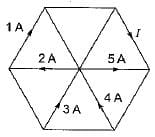Test: Basic Nodal & Mesh Analysis - 1 - Question 10

The value of dependent source for the circuit shown below is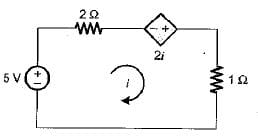Detailed Solution for Test: Basic Nodal & Mesh Analysis - 1 - Question 10

Applying KVL in the loop,
5 = 2i — 2i + i or i = 5 A
∴ Value of dependent source
= 2i = 2 x 5 = 10 volt

## GATE Electrical Engineering (EE) 2023 Mock Test Series

22 docs|274 tests
Information about Test: Basic Nodal & Mesh Analysis - 1 Page
In this test you can find the Exam questions for Test: Basic Nodal & Mesh Analysis - 1 solved & explained in the simplest way possible. Besides giving Questions and answers for Test: Basic Nodal & Mesh Analysis - 1 , EduRev gives you an ample number of Online tests for practice

## GATE Electrical Engineering (EE) 2023 Mock Test Series

22 docs|274 tests(Scan QR code)